# Differential Cryptanalysis Tutorial

Much has been written about this method of breaking ciphers. I've had trouble finding a concise, yet complete, tutorial on how to implement these attacks. I didn't really use a single reference while figuring out how this one works; instead I've done a lot of reading in different papers and eventually "got it". I think the existing literature tends to skim over the process of recovering keys and focuses much more on the differentials themselves. Here I'll try to do both subjects justice and explain how this technique works.

Source Code - This program, written in C, implements the simplified version of the toy cipher. It then calculates the most probable differential characteristics, displays all possible inputs/outputs for one selected characteristic (4 -> 7), and recovers the key in less time than brute force. I recommend following the code while reading this page; starting from main() and checking out the functions as this tutorial proceeds may speed you toward the "Eureka!" moment.

### The Target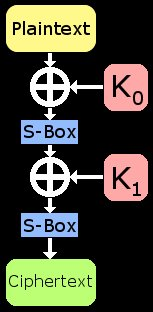Immediately, I'll describe a toy cipher that will be broken using this technique. It is a small and simple 2-round SPN that uses only substitution boxes and XOR key-mixing. The algorithm accepts a 4-bit block as input along with an 8-bit key; it outputs a 4-bit block. First the plaintext is XOR'd with the first 4 bits of the key (called subkey 0 or K0). Next this is fed into a 4-bit S-Box that provides the cipher's non-linearity. The output of the S-Box is then mixed via XOR with the second 4 bits of the key (subkey 1 or K1) and run though the S-Box again. The block size is small to facilitate learning and this keeps the S-Box size small as well. The parameters of this toy cipher are such that the reader could work through the process "by hand" on paper if desired.

We'll define the actual values in the S-Box later. For now, it is sufficient to say that the mapping is bijective. This means that for every input there is a unique output and every output also uniquely cooresponds to an input. In other words, if one knows the output of the S-Box, they can easily recover the cooresponding input to it. If this cipher only used S-Boxes, anyone could easily take advantage of this and run a ciphertext backward through the boxes to recover the plaintext. If the cipher used only XOR key-mixing, an attacker could use the properties of XOR and a single known plaintext/ciphertext pair to recover the key. By combining these functions, the security of this cipher is improved dramatically.

### Simplifying the Target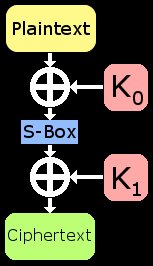It is often useful to reduce a cipher's schematic to a simpler form. By removing portions that aren't important, its sometimes easier to focus on the task at hand. Remember that the S-Box is entirely known to the attacker and is reversible. This means that if we know some ciphertext, we also know the inputs to the final S-Box. Thus, it provides no security, and can be ignored. If desired, one could make some very simple adaptions to the final attack to include the last S-Box. Once we acknowledge this fact, we are left with XOR->SBox->XOR. Even if we know a plaintext and cooresponding ciphertext block, the XORs "block" us from using basic algebra to determine the subkeys (K0 and K1).

Instinct might tell you that we need to test every key in K0 and every key in K1 until the correct key is found. In other words, the brute force time for this cipher is 28. First, note that for each block there is more bits of keying material than there are plaintext bits. This means that for known plaintext/ciphertext block, there is more than one key that will do the job. This multiple keys problem can create red herrings even during brute forcing. We'll assume that once an attacker wants to test a key, he'll test it against all known plaintext/ciphertext collected. If everything matches up, it is the correct key.

We do not need to test every single K0 and K1, however. If we make a guess at K0 and have some known plaintext/ciphertext blocks handy, basic algebra can be used to find the cooresponding guess at K1. To find this guess at K1, take your guess at K0 and XOR it with a known plaintext block. This provides the input to the S-Box; run it through. Use the output of the S-Box and XOR it with the cooresponding ciphertext block. This process provides a guess at K1. Now that you have a guess at both subkeys, do the test procedure and try them against all known pairs. If everything checks out, you found the key. This smarter brute forcing method only takes 24 guesses (thats 16 total!).

### Overview of the Attack

We're going to break this cipher after testing only 6 keys instead of 16. To do this, we'll need to not only know some plaintext/ciphertext; we need to choose it! Differential cryptanalysis is a chosen-plaintext attack. In this model, the attacker is able to make a cryptosystem encrypt data of his choosing using the target key (which is the secret). By analyzing the results that come back (the known ciphertext), the attacker can determine the key being used. Once the key is recovered in this way, future transmissions using it can be quickly decrypted. The chances of this attack model happening in the real-world are admittedly questionable, but it happens. The rise of technology, the internet, and automated data systems, makes this scenario far more likely than is expected at first glance.

### Differential Characteristics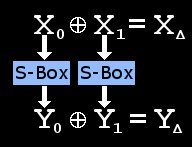Lets say you have a value X0 and a value X1; the XOR differential of X0 and X1 is simply X0 XOR X1. Look at the toy cipher's schematic for a moment; wouldn't it be cool if we knew the output value of that S-Box? We don't, but we can get some information about that value and exploit it. Differential characteristics have a very fun property; XORs do not affect them. We can't know the output of the S-Box because the key-mixing of K1 screws it up. This XOR key-mixing does not affect a differential of two values, however. The values themselves will change as they proceed through the XOR, but their relationship to each other will not. In math, it looks like this: X0 + X1 = (X0 + K1) + (X1 + K1). The only time this XOR differential gets changed is when the flow of data hits the S-Box.

Lucky for us, the S-Box is not perfect(which is impossible). A bit of statistical analysis will reveal what an input differential is likely to be transformed into when it goes through the S-Box. While we are figuring out what the output differential will be, also take note of all the possible input values (X0 and X1) that produce this transformation. Lets say we know a differential characteristic that has a high probability of holding true. For example, lets say that 6 out of 16 times, that two random inputs to the S-Box XOR together to produce the value 4. Additionally (6 out of 16 times), when these values are run through the S-Box and then XOR'd together, they will produce the differential value 7. We would say that the differential characteristic "4 --> 7" holds true 6/16 for that S-Box. Take note that there are only 6 actual values involved that can make this occurance happen; that fact will come into play later.

### Finding Characteristics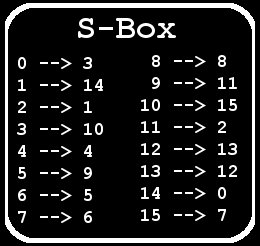The process of finding these differential characteristics is pretty straightforward. Simply examine every possible 4-bit input to the S-Box (X0) and XOR it with every other possible input to the S-Box (X1). The result of this XORing is called an input differential and the value found selects a row in the differential characteric table we're building. The column is selected by running each value (X0 and X1) through the S-Box and then XORing the outputs together. This value is called the output differential. Everytime these values create this input differential leading to an output differential increment the number (starting at 0 for all cells) in the table.

We are looking for differential characteristics that occur frequently (large numbers in the table). In other words, if two numbers are selected at random that satisfy the input differential; there is a high probability that the output differential will be satisfied as well. We don't need to know the actual random values to guess this if we can find a good characteristic to use. Once you've found a good characteristic, find all of the specific input values that produce it and their cooresponding output values. In our case, the characteristic "4 --> 7" has 6 such values (out 16 total). Make note of these values; they will help us find the key soon. One such value set is shown below.### Choosing the Plaintexts

Now that we've found a good strong differential characteristic that holds true often, we'll start the attack. Remember that this is a chosen-plaintext attack. We'll generate our known plaintext/ciphertext blocks in pairs. First, choose a plaintext block at random and call it P0. Next, XOR it with the input differential (4 in our case) from the chosen characteristic to produce P1. Now run each of these through the cipher (which is using the secret keys) to produce C0 and C1. You have just produced a chosen pair based on your differential characteristic. If you do this and C0 XOR C1 is equal to our chosen characteristic's output differential (7 in our case), then this is a good pair. Once you find one, you can stop choosing plaintext/ciphers. Store the other bad pairs, but set the good pair aside for the next step. The other pairs will be used to validate key guesses but normal known-plaintext can be used for this as well.

### Finding the Key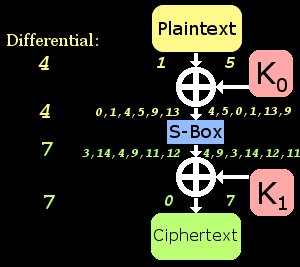Shown to the right is a diagram of an example good pair applied to the cipher. I didn't understand how differentials could be used to find the key is less than brute force time until I drew a similar diagram on paper. On the left side of the schematic, you'll see one of the plaintext/ciphertext known-blocks and on the right, the other. Notice that the plaintext blocks XOR together to produce a differential equal to the input diff(4) of our characteristic. Also notice that the ciphertext blocks XOR together similarly to produce the output differential of 7. This makes it a good pair and usable for us. What we don't know is the actual inputs and outputs of the S-Box. Remember that only 6 input value pairs can produce that "4 --> 7" characteristic. This means that those unknown values in the middle can only have one of those 6 pre-computed values!

I've listed all of the possible values (again pre-computed during our search for the characteristic) on the diagram. By guessing that one of S-Box inputs is 9, we are also guessing that its output is 11. Using some basic algebra, this makes our guess at K0 equal to 8 and our guess at K1 equal to 11. In this example, that is the correct key and I verified that by testing it against a bunch of other pairs (bad ones mostly). If this guess were not correct, then no big deal; there's only 5 more to try. The number of key guesses we need to make is equal to the probability of the characteristic holding true. In this case there are 6 possible keys because it held true 6/16 times. Using a characteristic with a prob. of 2 would make the search even shorter but it would be more difficult to find a usable good pair.

### Good Job!

It took some time, but I finally understand this technique and how to actually use it. If things are not clear, have an extra look at that last diagram; thats what did it for me. Realize that this attack will not recover the key; it will reduce the range of possible intermediate (and thus key) values down to number that is manageable. In this example, we found the key in 6 guesses instead of 16. The success of the attack as implemented in my code is completely dependent on finding a good pair. I modified it to run 100 times and count successful key recoveries. If 4 chosen-plaintext pairs were used, it found the key 75% of the time. If 8 pairs were used, it hovered around a 95% success rate. 100% key recovery was achieved at around 16 chosen-pairs. Check out the code also; it may help clarify some of this stuff.

As always, I welcome any comments on this article. If you have any thoughts on how I can improve it, please drop me an email. In the meantime, never lose that thirst for knowledge.

### A Paint-Mixing Analogy

In an effort to help the reader further understand how this works, I made the diagram below. It shows a good pair running through the cipher and the associated differentials along the way. The operation in GIMP/Photoshop that was used to "XOR" the colors was "Difference" because it has the same properties. The good differential characteristic used here is "Blue --> Purple". Some folks get along better with visuals than numbers and if you study this along with the code and the rest of this tutorial, it may help.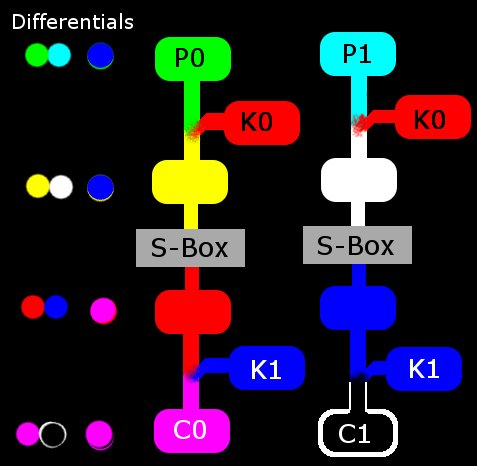### Extensions to the Attack

The known-plaintext attack model is more likely to occur in the real-world than the chosen-plaintext. For this reason, it would be nice to extend this attack to cover that possibility. The concept here is pretty simple and I'll implement it soon for posting here. Capture a bunch of plaintext/ciphertext pairs and compare them to each other. Stop collecting data when you find a good pair based on your chosen differential characteristic. So for example, if knownP5 XOR knownP34 is equal to 4 (our input differential) and knownC5 XOR knownC34 is equal to 7 (our output differential); that is a good pair! Finish the attack as it is described above and you have a known-plaintext variant of differential cryptanalysis. In the case of this 2-round toy cipher, you can even test for any of the characteristics that occur more than 0 times (there are 4 that occur 6/16 and are thus most likely). Remember though, the more testing you do on the actual data; the more time it is going to take. The goal is get that key faster than brute force so find the happy medium.

The differential attack can also be adapted for more than 2-rounds (and in the real world, it must be). The way to do this is by chaining differential characteristics together. If this cipher had 3 rounds, it would reduce to XOR->SBOX->XOR->SBOX->XOR. Note that "4 --> 7" holds true 6/16 and "7 --> 11" holds true 4/16. The plaintext/ciphertext pairs would be chosen such that the plaintext differential is equal to 4. A good pair would be one of these whose ciphertext differential is equal to 11. This relationship tells us that there is a reasonable probability that round 2 has a differential of 7. We follow this assumption and test the resulting 6 possible round 1 subkeys, 4 possible round 2 subkeys. This means that instead of testing 256 keys by brute force, we are testing 24 keys by differential cryptanalysis. Note: Round #1 may only require testing 4 of these subkeys but I am unsure of this. This adaptation will likely be part of the next tutorial I write here.